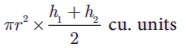Home | | Maths 10th Std | Volume of frustum of a cone

# Volume of frustum of a cone

Let H and h be the height of cone and frustum respectively, L and l be the slant height of the same.

Volume of frustum of a cone

Let H and h be the height of cone and frustum respectively, L and l be the slant height of the same.

If R, r are the radii of the circular bases of the frustum, then  volume of the frustum of the cone is the difference of the volumes of the two cones.Since the triangles ABC and ADE are similar, the ratio of their corresponding sides are proportional.Example 7.23 If the radii of the circular ends of a frustum which is 45 cm high are 28 cm and 7 cm, find the volume of the frustum.

### Solution :

Let h, r and R be the height, top and bottom radii of the frustum.

Given that, h = 45 cm, R = 28 cm, r = 7 cmTherefore, volume of the frustum is 48510 cm3

The adjacent figure represents an oblique frustum of a cylinder. Suppose this solid is cut by a plane through C, not parallel to the base AB, thenwhere h1 and h2 denote the greatest and least height of the frustum.

Then its volume =Tags : Definition, Formula, Solved Example Problems | Mensuration | Mathematics , 10th Mathematics : UNIT 7 : Mensuration
Study Material, Lecturing Notes, Assignment, Reference, Wiki description explanation, brief detail
10th Mathematics : UNIT 7 : Mensuration : Volume of frustum of a cone | Definition, Formula, Solved Example Problems | Mensuration | Mathematics